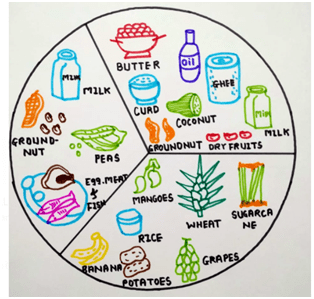# CBSE Diagrams for Class 6 Important for Exams

## CBSE Diagrams for Class 6

In class 6, students mostly get idea when any diagrams are there. The students come to understand the topic when it is diagrammatically explained. There are 9 chapter are included in class 6.  All the diagrams are drawn on the basis of this biology chapters. All the diagrams are important to understand the main concept of the biology chapters. This diagrams can be ask in the exams.

chapter 2: Components of food: The diagram is giving idea about sources of food. The food and sources of food is important.Figure 1: Sources of food

chapter 7: Getting to know plants: It is the diagram of plant and all the parts of plant. In the same chapter the flower diagram is also drawn, which gives information about all parts of the flower and location of all the parts of the flower along with this functions are also included in the chapter.Figure 2: Parts of a flowering plantFigure 3: The flower diagram

Chapter 8: Body movements: The 8th chapter in the syllabus is body movements. The body movement all can occur due to skeleton and 206 bones. Therefore the skeleton diagram is included which tell us about all the bones and their location in the body. By understanding the skeleton diagram students can easily observe the parts of the body also.Figure 4: Skeleton

Chapter 14: Water: This chapter contains all the importance of water in our day to day life and how recycling of the water occurs. For the better understanding of water recycling, water cycle diagram is important which gives complete idea about how recycling of the water occurs on an earth. Now a days many strategies are been going to save the water, along with that rain water harvesting is also vital. Many of the time students does not understand the term only from description instead they will get idea when they observe the diagram so rain water harvesting diagram is shown.Figure 5: The water cycleFigure 6: Rainwater harvesting

Chapter 15: Air around us

Due to more activities about pollution are taken, but along with this the chapter air is included in chapter 6. So student must know about the composition of air. Therefore students can understand which air is good for health and what types of gases are present in air. What is mean by fresh air and what is odorous air, so all the information can be understood through the chapter. For this purpose composition of air diagram is included in the chapter.Figure 7: Composition of air

These diagrams are included in class 6 biology chapters. The important diagrams has been drawn above.

Updated: December 3, 2021 — 12:11 am